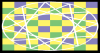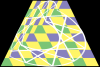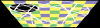# GMLDrawing Distorted Texture Primitives - Texture Folds Wrong

E

#### Ephemeral

##### Guest
My attempt at creating a surface-drawing script similar to draw_sprite_pos() has run into a snag.

Here is the script:
Code:
``````///@description draw surface perspective
///@param x
///@param y
///@param surface
///@param distort
///@param squish

var surface_id = argument2;
var distortion = argument3;
var squish = argument4;

// Vett Arguments

if (!surface_exists(surface_id)) return false;

var flat_width = surface_get_width(surface_id);
var flat_height = surface_get_height(surface_id);

// Vertex Placement

var tlx = (argument0 - (flat_width / 2)) + distortion; // Top Left
var trx = (argument0 + (flat_width / 2)) - distortion; // Top Right
var blx = (argument0 - (flat_width / 2)) - distortion; // Bottom Left
var brx = (argument0 + (flat_width / 2)) + distortion; // Bottom Right

var top_y = (argument1 - (flat_height / 2)) + squish; // Top
var bot_y = (argument1 + (flat_height / 2)) - squish; // Bottom

// Vertex Drawing

var texture_id = surface_get_texture(surface_id);
draw_primitive_begin_texture(pr_trianglestrip, texture_id);
draw_vertex_texture(tlx, top_y, 0, 0);
draw_vertex_texture(blx, bot_y, 0, 1);
draw_vertex_texture(trx, top_y, 1, 0);
draw_vertex_texture(brx, bot_y, 1, 1);
draw_primitive_end();

return true;``````
The result of
Code:
``draw_surface_perspective(x, y, srf_persp, 0, 256);``is as expected.

However, the result of
Code:
``draw_surface_perspective(x, y, srf_persp, 256, 0);``is this, which I am at a loss for how to fix.
How does GMLs built in draw_sprite_pos() handle this?

#### Bart

##### WiseBart
What you see makes sense. The surface can be seen as a square consisting of two triangles.
You change those triangles' dimensions but more importantly, also their shapes, without modifying the texture uvs accordingly. So the result becomes distorted.

Since you use a trianglestrip, the uvs on the diagonal already correspond between the two triangles. Which should be the case.
You probably have to apply the same transform that you apply to the vertices to the corner uv coordinates as well (the ones not on the diagonal), but instead applied to the range of [0,1].

Depending on the effect you're looking for, it may be easier to just use a perspective transformation while drawing this primitive, then reset the projection after that.
Although, in that case, you loose control over the exact location of the vertex coordinates.

E

#### Ephemeral

##### Guest
You change those triangles' dimensions but more importantly, also their shapes, without modifying the texture uvs accordingly.
I don't understand. There're only four vertexes, and the corners of the texture are exactly where they're supposed to be.

You probably have to apply the same transform that you apply to the vertices to the corner uv coordinates as well (the ones not on the diagonal), but instead applied to the range of [0,1].
This sounds like a really round-a-bout way to get the same effect as simply using draw_vertex() instead of draw_vertex_texture(), which would defeat the point. As I said, I'm trying to replicate the behavior of draw_sprite_pos().

just use a perspective transformation
This function is a perspective transfor --- oh, I'm guessing you mean go 3d? No thanks. I just want the texture not to break like that. How do I get it to remain intact while distorted?

#### NightFrost

##### Member
While I can't help with solution, I can tell the result you're seeing is called affine texture mapping. I recall it's been discussed here before, so if there's workarounds you may be able to find them with search.

E

#### Ephemeral

##### Guest
I found a couple other threads with with people attempting the same thing and having the same problem. "affine texture mapping" was a useful term, thanks

However, none of those threads had solutions either, so I'm still stuck.

E

#### Ephemeral

##### Guest
Alright, I decided to just brute force it with extra triangles.

I experimented with several variations, and this is the one that produced the best result.Code:
``````///@description draw surface perspective
///@param x
///@param y
///@param surface
///@param distort
///@param squish

var surface_id = argument2;
var distortion = argument3;
var squish = argument4;

// Vett Arguments

if (!surface_exists(surface_id)) return false;

var flat_width = surface_get_width(surface_id);
var flat_height = surface_get_height(surface_id);

var triangulation_count = 64;

// Vertex Placement

var tlx = (argument0 - (flat_width / 2)) + distortion; // Top Left
var trx = (argument0 + (flat_width / 2)) - distortion; // Top Right
var blx = (argument0 - (flat_width / 2)) - distortion; // Bottom Left
var brx = (argument0 + (flat_width / 2)) + distortion; // Bottom Right

var top_y = (argument1 - (flat_height / 2)) + squish; // Top
var bot_y = (argument1 + (flat_height / 2)) - squish; // Bottom

// Draw With Vertical Hatching

var texture_id = surface_get_texture(surface_id);
var tri_increment = 1 / triangulation_count;
draw_primitive_begin_texture(pr_trianglestrip, texture_id);

// Draw Left Half
for (var lv = 0; lv < 1; lv += tri_increment)
{
draw_vertex_texture(lerp(blx, argument0, lv), bot_y, lv / 2, 1);
draw_vertex_texture(lerp(tlx, argument0, lv), top_y, lv / 2, 0);
}
draw_vertex_texture(argument0, bot_y, 0.5, 1); // Center Point at Bottom
// Draw Right Half
for (var rv = tri_increment; rv <= 1; rv += tri_increment)
{
draw_vertex_texture(lerp(argument0, trx, rv), top_y, 0.5 + (rv / 2), 0);
draw_vertex_texture(lerp(argument0, brx, rv), bot_y, 0.5 + (rv / 2), 1);
}

draw_primitive_end();

return true;``````
The caveat is, of course, the inefficiency, but there's also weird behavior near the edges of the surface. I found that just making the surface 128 pixels bigger than the image in every dimension mostly fixed that, though.